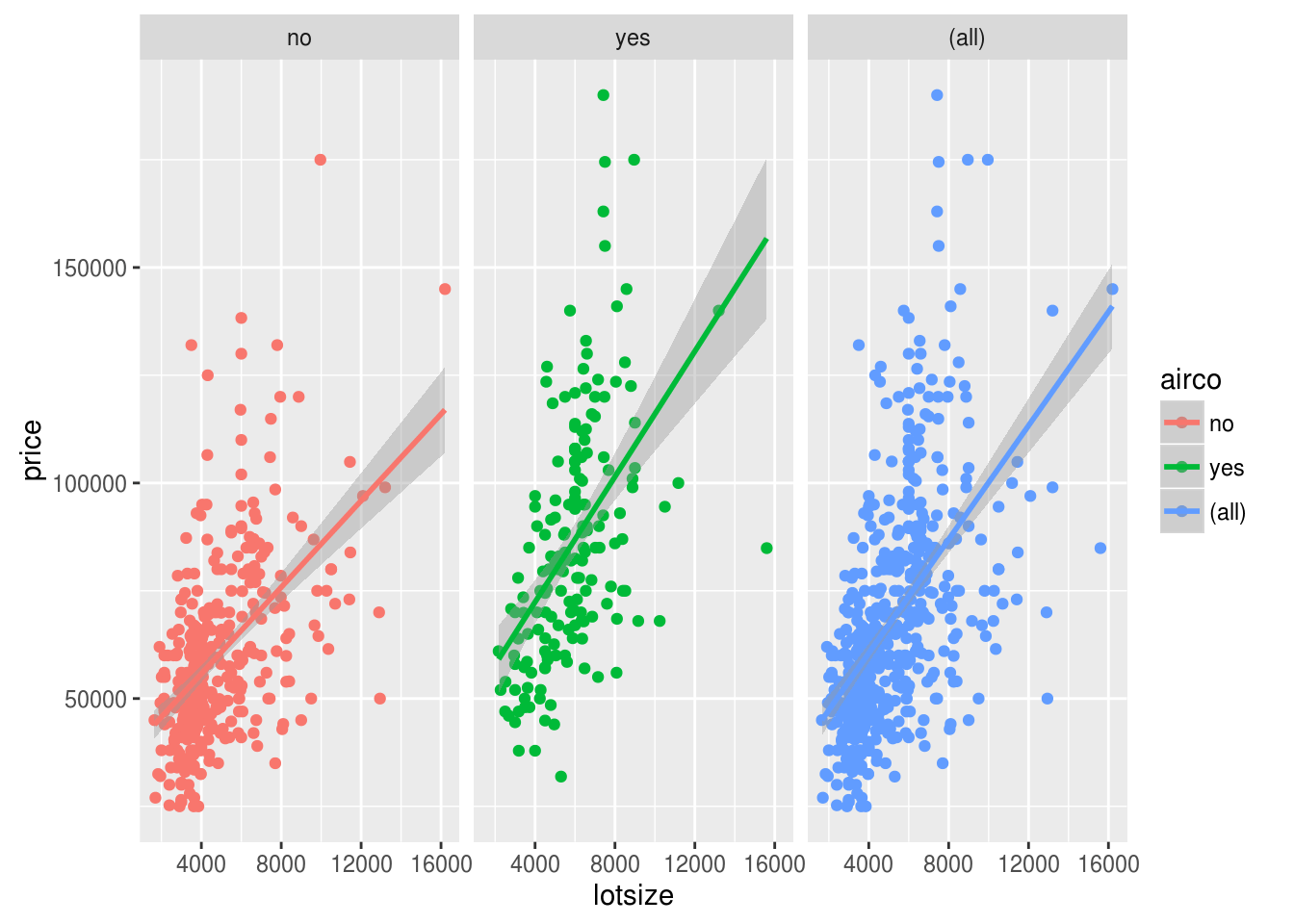# Linear Regression Lines and Facets in ggplot2

In this post, we will look at how to add a regression line to a plot using the “ggplot2” package. This is mostly a review of what we learned in the post on adding a LOESS line to a plot. The main difference is that a regression line is a straight line that represents the relationship between the x and y variable while a LOESS line is used mostly to identify trends in the data.One new wrinkle we will add to this discussion is the use of faceting when developing plots. Faceting is the development of multiple plots simultaneously with each sharing different information about the data.

The data we will use is the “Housing” dataset from the “Ecdat” package. We will examine how lotsize affects housing price when also considering whether the house has central air conditioning or not. Below is the initial code in order to be prepared for analysis

``library(ggplot2);library(Ecdat)``
``````## Loading required package: Ecfun
##
## Attaching package: 'Ecdat'
##
## The following object is masked from 'package:datasets':
##
##     Orange``````
``data("Housing")``

The first plot we will make is the basic plot of lotsize and price with the data being distinguished by having central air or not, without a regression line. The code is as follows

``ggplot(data=Housing, aes(x=lotsize, y=price, col=airco))+geom_point()``We will now add the regression line to the plot. We will make a new plot with an additional piece of code.

``ggplot(data=Housing, aes(x=lotsize, y=price, col=airco))+geom_point()+stat_smooth(method='lm')``As you can see we get two lines for the two conditions of the data. If we want to see the overall regression line we use the code that is shown below.

``ggplot()+geom_point(data=Housing, aes(x=lotsize, y=price, col=airco))+stat_smooth(data=Housing, aes(x=lotsize, y=price ),method='lm')``We will now experiment with a technique called faceting. Faceting allows you to split the data by various subgroups and display the result via plot simultaneously. For example, below is the code for splitting the data by central air for examining the relationship between lot size and price.

``ggplot(data=Housing, aes(lotsize, price, col=airco))+geom_point()+stat_smooth(method='lm')+facet_grid(.~airco)``By adding the “facet_grid” function we can subset the data by the categorical variable “airco”.

In the code below we have three plots. The first two show the relationship between lotsize and price based on central air and the last plot shows the overall relationship.

``ggplot(data=Housing, aes(lotsize, price, col=airco))+geom_point()+stat_smooth(method="lm")+facet_grid(.~airco, margins = TRUE)``By adding the argument “margins” and setting it to true we are able to add the third plot that shows the overall results.

So far all of are facetted plots have had the same statistical transformation of the use of a regression. However, we can actually mix the type of transformations that happen when facetting the results. This is shown below.

``ggplot(data=Housing, aes(lotsize, price, col=airco))+geom_point()+stat_smooth(data=subset(Housing, airco=="yes"))+stat_smooth(data=subset(Housing, airco=="no"), method="lm")+facet_grid(.~airco)``In the code we needed to use two functions of “stat_smooth” and indicate the information to transform inside the function. The plot to the left is a regression line with houses without central air and the plot to the right is a LOESS line with houses that have central air.

Conclusion

In this post, we explored the use of regression lines and advance faceting techniques. Communicating data with ggplot2 is one of many ways in which a data analyst can portray valuable information.